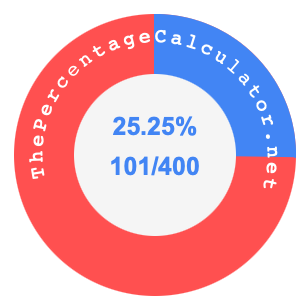25.25 percent as a fractionHere we will not only show you how to convert 25.25 percent to a fraction (25.25% as a fraction), but also illustrate 25.25 percent and 25.25 as a fraction on a pie chart.

Converting 25.25 percent to a fraction means that you want to convert 25.25% to a fraction with a numerator and denominator.

Percent means per hundred, so 25.25 percent means 25.25 per hundred. Thus, you can make 25.25 the numerator and 100 the denominator, and make 25.25 percent a fraction, like this:

25.25/100

The numerator and denominator in a fraction should not have a decimal point. You can eliminate the decimal point by multiplying both the numerator and denominator by 100 to get the following fraction:

2525/10000

The greatest common factor (GCF) of 2525 and 10000 is 25. Therefore, simplify the fraction above by dividing the numerator and deniminator by 25, like this:

2525 ÷ 25= 101
10000 ÷ 25 = 400

That's it! 25.25 percent as a fraction in its simplest form is displayed below:

25.25% = 101/400

The illustration below shows 25.25 percent and 25.25 as a fraction on a pie chart. As you now know, 25.25% is the same as 101/400.Percent to Fraction Converter
Here you can submit another percent that we can convert to a fraction.

25.26 percent as a fraction
Here is the next percent on our list that we converted to a fraction.